[Introduction]  [Signal arithmetic]  [Signals and noise]   [Smoothing]   [Differentiation]  [Peak Sharpening]  [Harmonic analysis]   [Fourier convolution]  [Fourier deconvolution]  [Fourier filter]   [Peak area measurement]  [Linear Least Squares]  [Multicomponent Spectroscopy]  [Iterative Curve Fitting]  [Hyperlinear quantitative absorption spectrophotometry] [Appendix and Case Studies]  [Peak Finding and Measurement]  [iPeak]   [iSignal]  [Peak Fitters]   [iFilter]  [iPower]  [List of downloadable software]  [Interactive tools]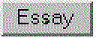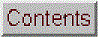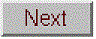## iFilter: interactive Fourier Filter, version 4.3

iFilter is Matlab implementation of a Fourier filter function for time-series signals, including interactive versions that allow you to adjust the filter parameters continuously while observing the effect on your signal dynamically. By adjusting the parameters, you can create lowpass, highpass, bandpass, band-stop (notch), comb pass, and comb reject filters with variable, frequency, width, and cut-off rate. The x-axis is labeled for time-based signals, where the independent variable is time in seconds, but the program can be used with any frequency axis (e.g. spacial frequency, etc). Click here to view or download iFilter.m.  You can also download it from the Matlab File Exchange.

### FouFilter.m: Basic Fourier Filter function

function ry=FouFilter(y, samplingtime, centerfrequency, frequencywidth, shape, mode)

Command-line Fourier filter function for time-series signal vector y; 'samplingtime' is the total duration of sampled signal in sec, millisec, or microsec; 'centerfrequency' and 'frequencywidth' are the center frequency and width of the filter in Hz, KHz, or MHz, respectively. If 'shape' = 1, the filter is Gaussian; as 'shape' increases, the filter shape becomes more and more rectangular (faster cut-off rate). Set 'mode' = 0 for band-pass filter, 'mode' = 1 for band-reject (notch) filter. Click here to view or download just this function.

Example: plot(FouFilter(tan(1:1000),15,2,2,0))

### iFilter.m: Keyboard-operated Interactive Fourier Filter (v 4.3, June, 2016)

iFilter is a keyboard-operated interactive Fourier filter function for time-series signal (x,y), with keyboard controls that allow you to adjust the filter parameters continuously while observing the effect on your signal dynamically. Optional input arguments set the initial values of center frequency, filter width, shape, plotmode (1=linear; 2=semilog frequency; 3=semilog amplitude; 4=log-log) and filter mode ('band-pass', 'low-pass', 'high-pass', 'band-reject (notch), 'comb pass', and 'comb notch'). In the comb modes, the filter has multiple bands located at frequencies 1, 2, 3, 4... times the center frequency, each with the same (controllable) width and shape.

The filtered signal can be returned as the function value, saved as a ".mat" file on the disk, or plays through the computer's sound system. Press K to list keyboard commands.  This is a self-contained Matlab function that does not require any toolboxes or add-on functions. Click here to view or download and place it in the Matlab path.

At the Matlab command prompt, type:  ifilter(x,y) or ifilter(y) or ifilter(xymatrix) or ry=ifilter(x,y,center,width,shape,plotmode,filtermode)

Version 4.3, June 2016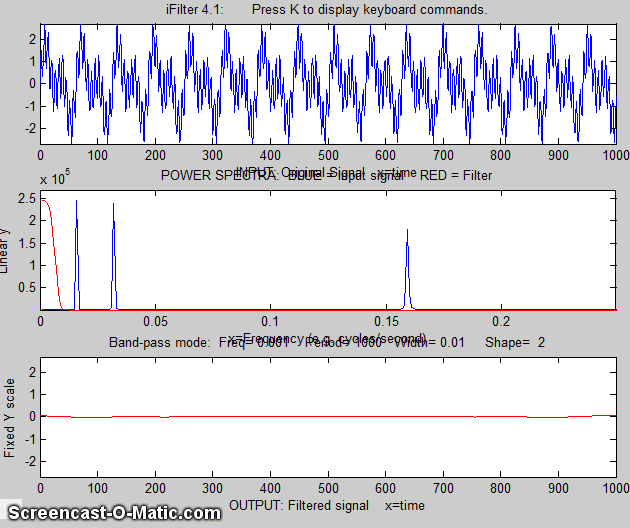Example 1:
Periodic waveform with 2 frequency components at 60 and 440 Hz.
x=[0:.001:2*pi];
y=sin(60.*x.*2.*pi)+2.*sin(440.*x.*2.*pi);
ifilter(x,y);

Example 2: uses optional input arguments to set initial values:
x=0:(1/8000):.3;
y=(1+12/100.*sin(2*47*pi.*x)).*sin(880*pi.*x)+(1+12/100.*sin(2*20*pi.*x)).*sin(2000*pi.*x);
ry=ifilter(x,y,440,31,18,3,'Band-pass');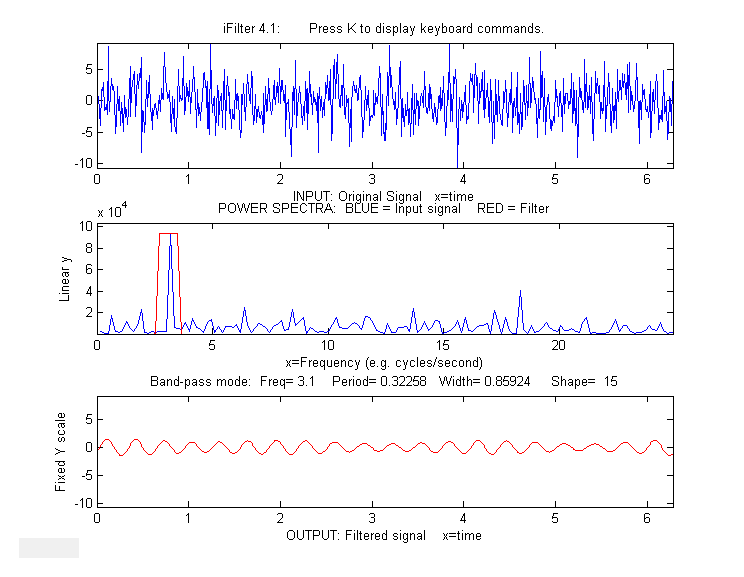Example 3: Picking one frequency out of a noisy sine wave.
x=[0:.01:2*pi]';
y=sin(20*x)+3.*randn(size(x));
ifilter(x,y,3.1,0.85924,15,1,'Band-pass');

Example 4: Square wave with band-pass vs Comb pass filter
t = 0:.0001:.0625;
y=square(2*pi*64*t);
ifilter(t,y,64,32,12,1,'Band-pass');
ifilter(t,y,48,32,2,1,'Comb pass');

Example 5:  MorseCode.m uses iFilter to demonstrate the abilities and limitations of Fourier filtering. It creates a pulsed fixed frequency (0.05) sine wave that spells out “SOS” in Morse code (dit-dit-dit/dah-dah-dah/dit-dit-dit), adds random white noise so that the SNR is very poor (about 0.1 in this example). The white noise has a frequency spectrum that is spread out over the entire range of frequencies; the signal itself is concentrated mostly at a fixed frequency (0.05) but the modulation of the sine wave by the Morse Code pulses spreads out its spectrum over a narrow frequency range of about 0.0004. This suggests that a Fourier bandpass filter tuned to the signal frequency might be able to isolate the signal from the noise. As the bandwidth is reduced, the signal-to-noise ratio improves and the signal begins to emerges from the noise until it becomes clear, but if the bandwidth is too narrow, the step response time is too slow to give distinct “dits” and “dahs”. The step response time is inversely proportional to the bandwidth. (Use the ? and " keys to adjust the bandwidth. Press 'P' or the Spacebar to hear the sound). You can actually hear that sine wave component better than you can see it in the waveform plot (upper panel), because the ear works like a spectrum analyzer, with separate nerve endings assigned to to specific frequencies, whereas the eye analyzes the graph spatially, looking at the overall amplitude and not at individual frequencies.  Watch an mp4 video of this script in operation, with sound. Also on YouTube.

Example 6:  This example shows a 1.58 sec duration audio recording of the spoken phrase "Testing, one, two, three", previously recorded at 44000 Hz and saved in WAV format (download link) and in ".mat" format (download link), loaded into iFilter, which is initially set to bandpass mode and tuned to a narrow segment that is well above the frequency range of most of the signal. It seems as if though this passband would miss most of the frequency components in the signal, yet even in this case the speech is intelligible, demonstrating the remarkable ability of the ear-brain system to make do with a highly compromised signal. Press P or space to hear the filter's output. Different filter settings will change the timbre of the sound.

>> v=wavread('TestingOneTwoThree.wav');
>> time=0:1/44001:1.5825;
>> waveform=v(:,2);
>> ifilter(time,waveform,1267,700,10,2,'Band-pass');

iFilter 4.3 KEYBOARD CONTROLS when figure window is topmost:

Adjust filter frequency.......Coarse (10% change): < and >
Fine
(1% change): left and right cursor arrows
Adjust filter width...........Coarse
(10% change): / and "
Fine
(1% change): up and down cursor arrows
Filter shape..................A,Z (A more rectangular, Z more Gaussian)
Filter mode...................B=bandpass; N or R=notch (band
reject);H=High-pass;
L=Low-pass; C=Comb pass; V=Comb notch.
Select plot mode..............1=linear; 2=semilog frequency
3=semilog amplitude; 4=log-log
Print keyboard commands.......K  Pints this list
Print filter parameters.......Q or W Prints ifilter with input
arguments: center, width, shape,
plotmode, filtermode
Print current settings........T  Prints list of current settings
Switch SPECTRUM X-axis scale..X switch between frequency and period
on the horizontal axis
Switch OUTPUT Y-axis scale....Y switch
output plot between fixed or
variable vertical axis.
Play output as sound..........P or Enter
Save output as .mat file......S

This page is part of "A Pragmatic Introduction to Signal Processing", created and maintained by Prof. Tom O'Haver , Department of Chemistry and Biochemistry, The University of Maryland at College Park. Comments, suggestions and questions should be directed to Prof. O'Haver at toh@umd.edu.Last updated: March, 2018. Number of unique visits to this site since May 17, 2008: